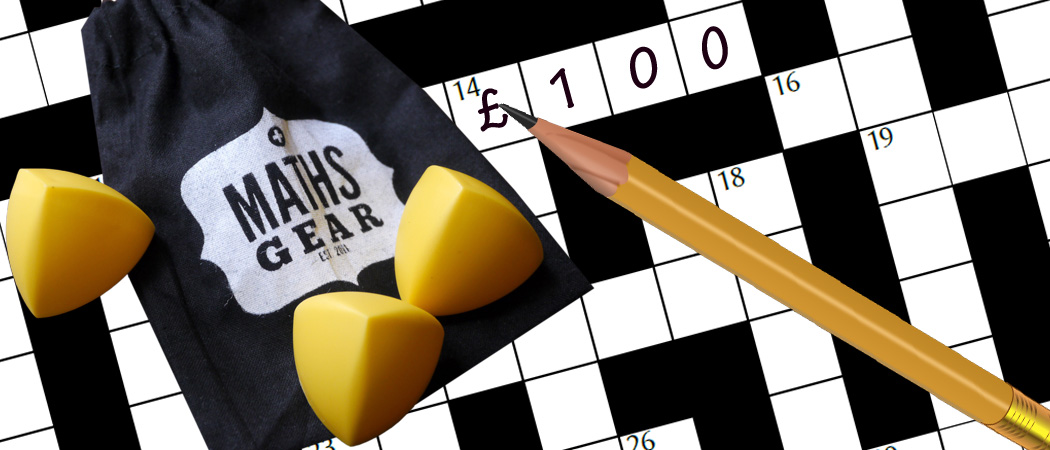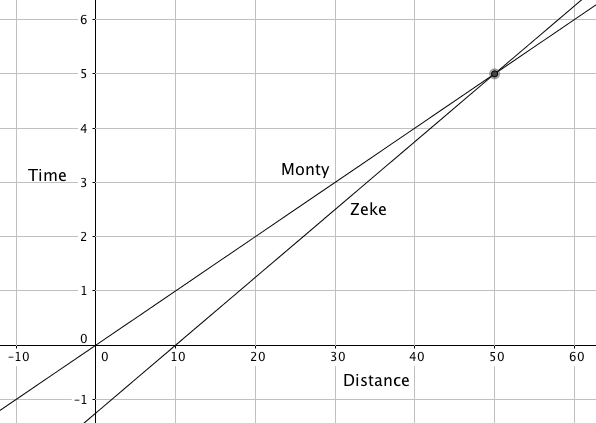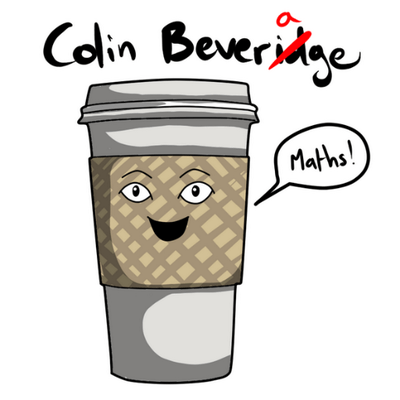# Crossnumbers and cross products

A crossnumber clue takes us plunging headfirst towards projective geometryOnce upon a time, there was a Chalkdust crossnumber clue:

27D The number of straight lines that go through at least two points of a 10 × 10 grid of points. (4)

Obviously, I could have looked that up on the OEIS, but that is not how I roll with the crossnumber. That’s tantamount to letting Humbug Scroggs win.

Instead, I wrote some code to do it. And in the process, stumbled across something a bit special. Or, as my MathsJam talk on the matter had it, a bit… Specials.The Specials. Image: WikiMedia Commons user Robman94, CC BY-SA 3.0.

## You’ve done too much, too much C1

What I needed was a way to give each line a unique reference, so I could avoid double-counting them. Since my previous student had asked me why we would ever write a line in the form $ax + by + c = 0$ (answer: beats me, but it’s as good as any other), that was on my mind: what if I simply indexed each line as a vector $(a,b,c)$?

That’s good, but it’s not quite good enough: any multiple of $(a,b,c)$ gives the same straight line, but there are several possible fixes for that. The simplest fix is to rescale it as a unit vector: this is what I did in my code. (Alternatives include scaling the vector to make the $z$-component 1, which requires special handling if $c=0$ and who can be bothered with that, at least at this stage? and—because we’re dealing with integers here, just making sure $a$, $b$ and $c$ are coprime. Even that runs into sign problems if you’re as lazy as I am, though.)

Once I’d merrily filled in 4492 in the grid, instead of getting on with the important business of doing the rest of the clues and cursing at Scroggs, something caught in my mind: if lines can be written as vectors… where does that lead?

## A message to you, 2D

My first question was: given two lines, $ax + by + c = 0$ and $px + qy + r = 0$, can I use the vectors $(a,b,c)$ and $(p,q,r)$ to find where the lines cross?

In my talk, I employed the well-known proof by MathsJam technique: "obviously it’s valid, or else I wouldn’t be talking about it." However, I know of no such result for proof by Chalkdust, and I can hardly ask you to take me at my word when I say "yes, you can: to find out where the lines cross, you find the cross product (and scale the result so the $z$-coordinate is 1)."

Can’t I at least leave this as an exercise for the re… no? Sheesh, tough editors. Fine.

Doing the cross product,$\begin{pmatrix}a \\ b \\ c\end{pmatrix} \times \begin{pmatrix} p \\ q \\ r\end{pmatrix} = \begin{pmatrix} br-cq \\ cp-ar \\ aq-bp\end{pmatrix},$and scaling it down gives $\left(\frac{br-cq}{aq-bp}, \frac{cp-ar}{aq-bp}, 1\right).$

Multiplying the equation of the first line by $p$ and the equation of the second by $a$ gives $apx + bpy + cp = 0$ and $apx +qay + ar = 0$, which leads to $bpy + cp = qay + ar$ and $(aq-bp)y = cp—ar$, so $y = \frac{cp-ar}{aq-bp}$. A similar process gives $x = \frac{br-cq}{aq-bp}$, as required. $\blacksquare$.

## It’s working for the rat race

I know I’ve proved it, but it’s worth showing that it works as well. Suppose we’ve got the following question, involving two rats, Zeke and Monty:Using a graph to find where Zeke catches Monty.

• Zeke scampers at 10m/s
• Monty scampers at 8m/s
• Zeke gives Monty a 10m head start
• How long does it take Zeke to catch up?

We can set that up as a pair of equations: $x-10t=0$ and $x-8t-10=0$, and convert them to the vectors $(1,-10,0)$ and $(1,-8,-10)$. Their vector product is $(100,10,2)$, which scales down to $(50,5,1)$; after 5 seconds, Zeke catches up with Monty, and the fact that this happens 50m from where Zeke starts is a freebie.

## The line through C & AC&A, as seen in the UK pre 2001. Image: Stuart Caie, CC BY 2.0.

We’re only starting to scratch the surface, though. If $(a,b,c)$ corresponds to a line and $(x,y,1)$ corresponds to a point, what happens if you take the cross product of the vectors corresponding to two points?

Surprise! You get a vector corresponding to the line connecting them. For example, if point $A$ is at (3,4) and point $C$ at (5,1), we’d ordinarily figure out a gradient and plug it into a formula leading us to $3x+2y-17=0$.

However, if we take the cross product of $(3,4,1)$ and $(5,1,1)$, we get $(3,2,-17)$, which corresponds—as one would hope—to the same line.

## Abstract jungle

So, what’s going on here?

One way of looking at it is to consider what’s happening in three dimensions—and in particular, where certain lines and planes intersect the $z=1$ plane.

We’ve glossed over, with a great deal of hand-waving, the idea that we can simply take the cross product of the vectors corresponding to two lines and scale the result to have $z=1$. Let’s firm that up a little bit: a point in our system corresponds to a line through the origin; it is represented in 2D coordinates by the $x$ and $y$ values where the line crosses the $z=1$ plane.

A line, meanwhile, is represented by a plane in this system. Its 2D representation is the intersection between the plane and $z=1$.

Now things start to drop out: any two points in the $z=1$ plane lie on a unique plane that also contains the origin. The normal vector of this plane is perpendicular to the vector from the origin to either of the points, so their cross-product will immediately give the normal of the plane, which corresponds to the line in the $z=1$ connecting them.

Similarly, any line in the $z=1$ plane lies on a unique plane through the origin; the line of intersection of two such plane is a line through the origin perpendicular to both normals, which again comes down to the cross product. Where that intersection line crosses the $z=1$ plane is the point of intersection of the original lines.

## The dawning of a new error

More astute readers will have spotted a hole in this: what happens if you take the cross product of two parallel lines? Something interesting, that’s what.

Take, for example, the parallel lines corresponding to $(3,2,-17)$ and $(3,2,1)$. Their cross product is $(36,-54,0)$—and it’s clearly not possible to scale that to meet the $z=1$ plane. If you’re a strict Euclidean, you dust your hands and say "They don’t intersect!"

If you’re more open-minded, you can say "there’s nothing wrong with the line through the origin and $(36,-54,0)$—why can’t it correspond to a point?" Answer: it can! We can associate each direction with a point at infinity—which opens up yet another tool for us to exploit.

For instance, if you know you have (in regular 2D) a line through (6,7) with a gradient of $-\frac{2}{3}$, you can find its equation by taking the cross product of $(6,7,1)$ and $(1,-\frac{2}{3},0)$ (the point at infinity corresponding to that gradient), giving you $(\frac{2}{3},1,-11)$, or $2x+3y-33=0$.

The points at infinity, of course, have lines corresponding to them: they turn out to be exactly the lines through the origin. Indeed, there’s even a line that goes through all of the points at infinity; it corresponds to the vector $(0,0,1)$.

## It’s up to you

Readers, I have to break it to you: we have been dabbling in projective geometry—and in particular, in the principle of duality, neither of which I’ll go into in detail here (you have a Google, correct? Excellent.)

There are all manner of projective peculiarities you can explore—you might like to consider a point/line as a sort of spinning-top shape on a unit sphere, or to consider higher dimensions if you want to take it one step beyond—but that way lies madness.

If this post has inspired you to attempt the Chalkdust crossnumber, you can find the latest one here. You can win a collection of prizes from Maths Gear if you send in a correct answer before 22 July 2017.Colin is a mathematician with nothing to prove. To prove this, he has written several books including Maths for Dummies, writes a blog, and appears in a monthly podcast.
@icecolbeveridge    www.flyingcoloursmaths.co.uk/    + More articles by Colin